•#### Learning Bayesian Models with R#### Overview of this book

Learning Bayesian Models with RCreditswww.PacktPub.comPrefaceFree Chapter
Introducing the Probability TheoryThe R EnvironmentIntroducing Bayesian InferenceMachine Learning Using Bayesian InferenceBayesian Regression ModelsBayesian Classification ModelsBayesian Models for Unsupervised LearningBayesian Neural NetworksBayesian Modeling at Big Data ScaleIndex## The Bayesian logistic regression model

The name logistic regression comes from the fact that the dependent variable of the regression is a logistic function. It is one of the widely used models in problems where the response is a binary variable (for example, fraud or not-fraud, click or no-click, and so on).

A logistic function is defined by the following equation: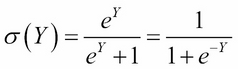It has the particular feature that, as y varies from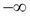to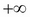, the function value varies from 0 to 1. Hence, the logistic function is ideal for modeling any binary response as the input signal is varied.

The inverse of the logistic function is called logit. It is defined as follows: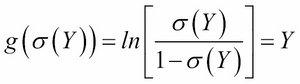In logistic regression, y is treated as a linear function of explanatory variables X. Therefore, the logistic regression model can be defined as follows: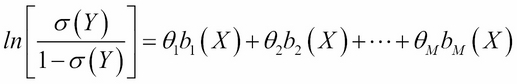Here,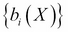is the set of basis functions and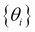are the model parameters as explained in the case of linear regression in Chapter 4, Machine Learning Using Bayesian Inference. From the definition of GLM in Chapter...